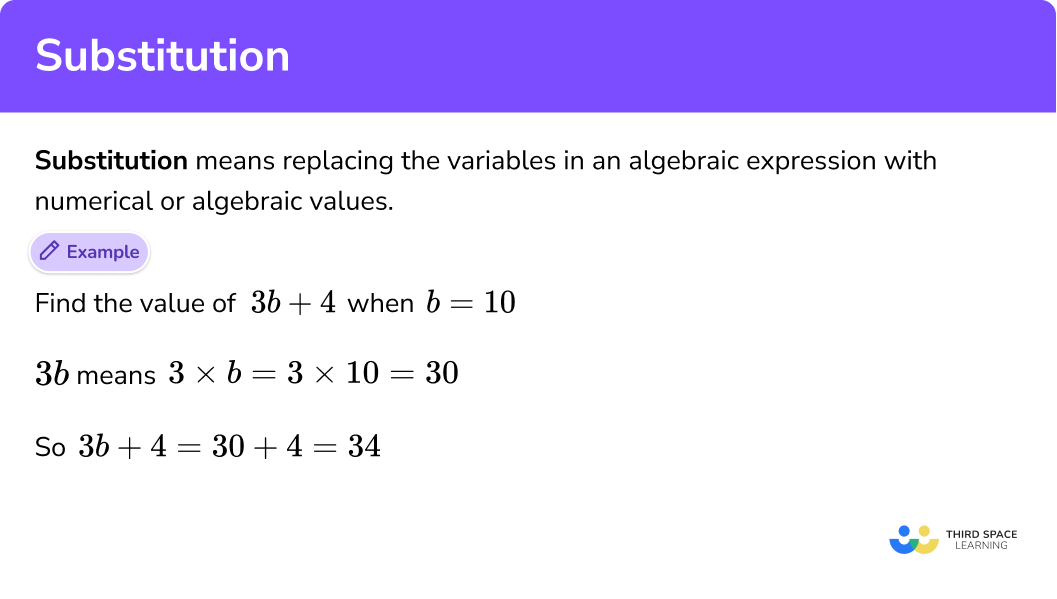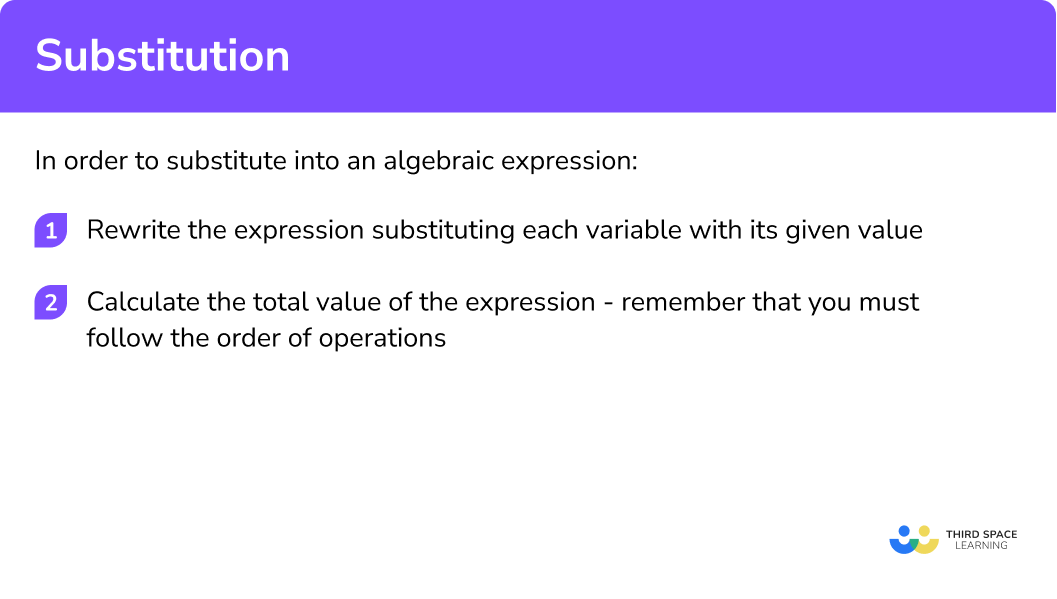# Substitution

Here is everything you need to know about substitution for GCSE maths (Edexcel, AQA and OCR). You’ll learn how to substitute positive numbers, negative numbers and decimals into various algebraic expressions.

Look out for the substitution worksheets and exam questions at the end.

## What is substitution?

Substitution means replacing the variables (letters) in an algebraic expression with their numerical values. We can then work out the total value of the expression.

We can substitute values into formulae to help us work out many different things. Examples range from the formula for the area of a triangle:

$A=\frac{1}{2} b h$

to the formula for calculating BMI:

$B M I=\frac{w}{h^{2}}$

### What is substitution?This lesson is part of our series on algebraic expressions. You may find it helpful to start with the main algebraic expressions lesson for a summary of what to expect and then also work through the following:

## How to substitute a value into an algebraic expression

In order to substitute into an algebraic expression:

1. Rewrite the expression substituting each letter with its given numerical value.
2. Calculate the total value of the expression. Remember that you must apply BIDMAS.

### Explain how to substitute a value into an algebraic expression in 2 steps## Substitution examples

### Example 1: basic expression, one variable

Find the value of 3b + 4 when b = 10.

1. Here b = 10 so we substitute the b in 3b + 4 for 10.

$3\times 10 + 4$

Remember that 3b means 3 ✕ b.

2We can now work out the value of the expression:

$3\times 10 + 4 = 34$

### Example 2: basic expression, one variable

Find the value of

$20-\frac{m}{5}$

when m = 35.

$20-\frac{m}{5}$
\begin{aligned} 20-\frac{35}{5} \end{aligned}

Remember that

$\frac{35}{5}$

means 35 ÷ 5.

\begin{aligned} 20-\frac{35}{5} &=20-7 \\ &=13 \end{aligned}

### Example 3: basic expression, two variables

Find the value of 3x − 5y when x = 5 and y = 4.

$3\times 5 – 5\times 4$

\begin{aligned} 3 \times 5-5 \times 4 &=15-20 \\ &=-5 \end{aligned}

Notice that we have applied BIDMAS – multiplying is done before subtracting.

### Example 4: brackets

Find the value of 2p(q − r) when p = 5, q = 15 and r = 6.

$2\times 5\left ( 15-6 \right )$

\begin{aligned} 2 \times 5 \times(15-6) &=2 \times 5 \times 9 \\ &=90 \end{aligned}

### Example 5: powers

Find the value of

$s^{2}(20-\sqrt{t})$

when s = 2 and t = 9.

$2^{2}(20-\sqrt{9})$

\begin{aligned} 2^{2} \times(20-\sqrt{9}) &=2^{2} \times(20-3) \\ &=2^{2} \times 17 \\ &=4 \times 17 \\ &=68 \end{aligned}

## Substituting decimals and negative numbers

We substitute decimals in the same way as any other number.

When substituting negative numbers we need to be particularly careful and remember the rules for operations with negatives.

In particular, remember that:

+ + = +
+ − = −

− − = +
− + = −

So 7 + − 3 = 4 and 6 − − 5 = 11.

These rules also apply for multiplying and dividing negative numbers.

Another important point to remember is that squared means multiplied by itself.

\begin{aligned} (-5)^{2} &=-5 \times-5 \\ &=25 \end{aligned}

Notice that when you square a negative number you get a positive answer.

### Example 6: decimals

Find the value of 4g(10 − 2h) when g = 2.5 and h = 1.8.

$4\times 2.5(10-2\times 1.8)$

\begin{aligned} 4 \times 2.5 \times(10-2 \times 1.8) &=4 \times 2.5 \times(10-3.6) \\ &=4 \times 2.5 \times 6.4 \\ &=64 \end{aligned}

### Example 7: negative numbers

Find the value of 5z2 + 7y when z = − 10 and y = − 3.

$5\times (-10)^{2} +7\times -3$

We have put the − 10 in brackets here as we need to make sure that we are squaring − 10 and not subtracting 10 squared.

\begin{aligned} 5 \times(-10)^{2}+7 \times-3 &=5 \times 100+7 \times-3 \\ &=500+-21 \\ &=479 \end{aligned}

### Example 8: negative numbers

Find the value of

$\frac{4 p\left(3 q^{2}-70\right)}{6+p}$

when p = − 4 and q = − 5.

$\frac{4 \times-4\left(3 \times(-5)^{2}-70\right)}{6+-4}$

\begin{aligned} \frac{4 \times-4\left(3 \times(-5)^{2}-70\right)}{6{+-4}} &=\frac{4 \times-4(3 \times 25-70)}{6+-4} \\ &=\frac{4 \times-4 \times 5}{6+-4} \\ &=\frac{-80}{2} \\ &=-40 \end{aligned}

## Using formulae

We substitute into formulae in exactly the same way. We use formulae to work different things out.

### Example 9: formulae

Speed is calculated using the formula

$S=\frac{D}{T}$

where D is distance and T is time.

Find the speed at which a car travelled if it took 2 hours to travel a distance of 100 miles.

Substituting into the formula:

$S=\frac{100}{2}$

Notice that we leave the S on the left-hand side.

$S=50mph$

### Example 10: formulae

$x=\frac{-b+\sqrt{b^{2}-4 a c}}{2 a}$

or

$x=\frac{-b-\sqrt{b^{2}-4 a c}}{2 a}$

can be used to solve quadratic equations.

Find the two possible values of x when a = 1, b = − 2 and c = − 15.

$x=\frac{2+\sqrt{(-2)^{2}-4 \times 1 \times-15}}{2 \times 1} \quad \text { or } \quad x=\frac{2-\sqrt{(-2)^{2}-4 \times 1 \times-15}}{2 \times 1} \mid$

$\begin{array}{l} x=\frac{2+\sqrt{(-2)^{2}-4 \times 1 \times-15}}{2 \times 1} \\ x=\frac{2+\sqrt{4–60}}{2} \\ x=\frac{2+\sqrt{64}}{2} \\ x=\frac{2+8}{2} \\ x=\frac{10}{2} \\ x=5 \end{array}$

$\begin{array}{l} x=\frac{2-\sqrt{(-2)^{2}-4 \times 1 \times-15}}{2 \times 1} \\ x=\frac{2-\sqrt{4–60}}{2} \\ x=\frac{2-\sqrt{64}}{2} \\ x=\frac{2-8}{2} \\ x=\frac{-6}{2} \\ x=-3 \end{array}$

### Common misconceptions

These are some of the common misconceptions around substitution:

• The meaning of ab

Remember in algebraic expressions, ab means a multiplied by b, not the number b written after the number a. So if a = 3 and b = 5, ab = 3 ✕ 5, not 35.

• Not applying BIDMAS

BIDMAS rules need to be followed here as in normal numerical calculations.

• Mistakes with negative numbers

The most common mistake is thinking that a negative number squared gives a negative answer. Remember that squared means multiplied by itself and a negative number multiplied by a negative number gives a positive answer.

### Practice substitution questions

1. Find the value of 7x-10 when x=5

65-325-57x-10=7 \times5-10=35-10=25

2. Find the value of \frac{y}{4}+8 when y=20

2832137\frac{y}{4}+8=\frac{20}{4}+8=5+8=13

3. Find the value of 7p-2q when p=8.5 and q=1.5

-6.556.51757p-2q=7(8.5)-2(1.5)=59.5-3=56.5

4. Find the value of 6g(3h-9) when g=10 and h=5

3606306066g(3h-9)=6(10)\times(3(5)-9)=60\times(15-9)=60\times6=360

5. Find the value of \frac{m^{2}+\sqrt{n}}{10} when m=8 and n=36

1074.444\frac{m^{2}+\sqrt{n}}{10}=\frac{8^{2}+\sqrt{36}}{10}=\frac{64+6}{10}=\frac{70}{10}=7

6. Find the value of 3e^2+8 when e= -4

-40562443e^{2}+8=3(-4)^{2}+8=3(16)+8=56

7. Force can be calculated using the formula F = ma where m = mass and a = acceleration. Calculate the force on an object with a mass of 2kg and an acceleration of 3m/s^{2} .

5 Newtons9 Newtons8 Newtons6 NewtonsF=ma\\
F=2\times3\\
F=6

8. Kinetic energy can be calculated using the formula KE=\frac{1}{2} m v^{2} , where m = mass and v = velocity. Calculate the kinetic energy of an object with mass 5kg moving at a velocity of 4m/s .

10 Joules40 Joules20 Joules100 JoulesKE=\frac{1}{2} m v^{2}\\
KE=\frac{1}{2}\times 5\times 4^{2}\\
KE=\frac{1}{2}\times5 \times 16\\
KE=40

### Substitution GCSE questions

1. Work out the value of 3x + 2y when x = 6 and y = 4

(1 mark)

3\times6 + 2 \times4 = 26

(A1)

2. Work out the value of \frac{4 a-10 b}{5} when a = 5 and b = − 2

(2 marks)

4\times5 = 20 and 10 \times− 2 = − 20

(M1)

\frac{20–20}{5}=\frac{40}{5} = 8

(A1)

3. The cost, C , of hiring a canoe is given by the formula

C = 20 + 2.5h

where h is the number of hours the canoe is to be hired for.

Calculate the cost of hiring the canoe for 6 hours.

(1 mark)

C = 20 + 2.5\times 6

(M1)

C = 20 + 15

C = 35

(A1)

## Learning checklist

• Substitute positive numbers, negative numbers and decimals into basic algebraic expressions
• Substitute into expressions involving brackets and powers
• Substitute into formulae

## Still stuck?

Prepare your KS4 students for maths GCSEs success with Third Space Learning. Weekly online one to one GCSE maths revision lessons delivered by expert maths tutors.

Find out more about our GCSE maths tuition programme.